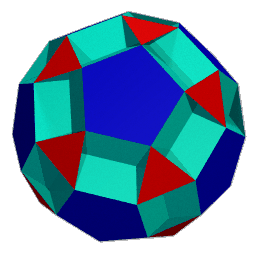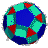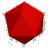# The Uniform Polyhedra

## Introduction

Uniform polyhedra consist of regular faces and congruent vertices. Allowing for non-convex faces and vertex figures, there are 75 such polyhedra, as well as 2 infinite families of prisms and antiprisms. A recently discovered uniform way of computing their vertex coordinates [Harel93] is the basis for a program to display all of these solids, among which are many beautiful and stunning shapes.

This text is an excerpt of Chapter 9 of R.  Maeder's book The Mathematica Programmer II. An expanded version of these Web pages can also be found in the Mathematica notebook `Polyhedra.ma` from the Illustrated Mathematics CD-ROM.

## About the Images on These Pages

The metric properties and graphics data were computed with a Mathematica program, developed by R. Maeder, based on a C program by Zvi Har'El.

The ray-traced images on these pages were rendered with POV-ray, from data computed with the program mentioned above, using a conversion program from Mathematica graphics format to POV-ray input.

### Programs and Images are Available!The Mathematica programs to compute and render the polyhedra are included on the CD-ROM that comes with The Mathematica Programmer II. The book contains also high-resolution color images of all uniform polyhedra. Follow the link to the book's home page for more information and direct ordering in association with amazon.com.

### Graphic Resources

There is one page for each polyhedron with a high-resolution image and geometrical information. The pages can be accessed in these ways:A visual index (sensitive map) of all 80 polyhedraList and thumbnail pictures of all Uniform PolyhedraA list sorted by Wythoff symbolA guided tour of all 80 polyhedra starts here

#### Animations

See the polyhedra spin about a symmetry axis for better visualization. The animations are linked through the high-resolution images on the individual polyhedra pages.

## Geometric Properties

### Symmetry Group

This listing indicates which type of symmetry the polyhedron has: dihedral, tetrahedral, octahedral, and icosahedral. The listing does not indicate whether the polyhedron possesses full (reflective) symmetry, or only rotational symmetry (most snub polyhedra).

### Vertex Configuration

The vertex configuration is the sequence of faces arranged around a vertex. Since vertices are congruent, this sequence is the same for all vertices. A regular n-sided polygon (an n-gon) is described by n. Star polygons are described by n/d, where n is the number of vertices, connected d apart. For example, 5/2 is the pentagram. Some polyhedra contain retrograde faces. For example, 4/3 is a square, traversed in the opposite direction. A regular 2-gon is degenerate and can be left out if it occurs in the formulae.You may also want to have a look at the collection of all stellated icosahedra.Programs and high-resolution images for uniform polyhedra are available in the book The Mathematica Programmer II by R. Maeder.All 75 uniform polyhedra, with background information, a clickable map, and animations.

A service provided by MathConsult AG, http://www.mathconsult.ch/.